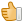#美玲的期中考試,國語、數學和社會的平均為91分，已知國語96分，數學比社會少5分，社會考幾分？

0 投票
198 浏览## 2 个回答

0 投票

91×3=273

273-96=177

177+5=182

182÷2=91

数学81分

273÷3=91（平均分）

（答案没错）
0 投票

91×3=273
273-96=177
177+5=182
182÷2=91

86+91+96=273
273÷3=91（平均分）